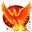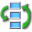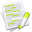#wx.AffineMatrix2DBase¶

A 2x3 matrix representing an affine 2D transformation.

This is an abstract base class implemented by wx.AffineMatrix2D only so far, but in the future we also plan to derive wx.GraphicsMatrix from it.

New in version 2.9.2.

##Class Hierarchy¶Inheritance diagram for class AffineMatrix2DBase:

##Known Subclasses¶

wx.AffineMatrix2D

##Methods Summary¶

 __init__ Default constructor. Concat Concatenate this matrix with another one. Get Get the component values of the matrix. Invert Invert this matrix. IsEqual Check that this matrix is identical with t. IsIdentity Check if this is the identity matrix. Mirror Add mirroring to this matrix. Rotate Add clockwise rotation to this matrix. Scale Add scaling to this matrix. Set Set all elements of this matrix. TransformDistance Applies the linear part of this matrix, i.e. without translation. TransformPoint Applies this matrix to the point. Translate Add the translation to this matrix. __ne__ Check that this matrix differs from t. __eq__ Check that this matrix is identical with t.

##Class API¶

class wx.AffineMatrix2DBase(object)

Possible constructors:

AffineMatrix2DBase()


A 2x3 matrix representing an affine 2D transformation.

### Methods¶

__init__(self)

Default constructor.

The matrix elements are initialize to the identity matrix.

Concat(self, t)

Concatenate this matrix with another one.

The parameter matrix is the multiplicand.

Parameters

t (wx.AffineMatrix2DBase) – The multiplicand.

#           | t.m_11  t.m_12  0 |   | m_11  m_12   0 |
# matrix' = | t.m_21  t.m_22  0 | x | m_21  m_22   0 |
#           | t.m_tx  t.m_ty  1 |   | m_tx  m_ty   1 |


Get(self)

Get the component values of the matrix.

Return type

tuple

Returns

( mat2D, tr )

Invert(self)

Invert this matrix.

If the matrix is not invertible, i.e. if its determinant is 0, returns False and doesn’t modify it.

#           | m_11  m_12  0 |
# Invert    | m_21  m_22  0 |
#           | m_tx  m_ty  1 |

Return type

bool

IsEqual(self, t)

Check that this matrix is identical with t.

Parameters

t (wx.AffineMatrix2DBase) – The matrix compared with this.

Return type

bool

IsIdentity(self)

Check if this is the identity matrix.

Return type

bool

Mirror(self, direction=HORIZONTAL)

Parameters

direction (int) – The direction(s) used for mirroring. One of wx.HORIZONTAL, wx.VERTICAL or their combination wx.BOTH.

Rotate(self, cRadians)

Add clockwise rotation to this matrix.

Parameters

Scale(self, xScale, yScale)

Parameters
• xScale (wx.Double) – Scaling in x direction.

• yScale (wx.Double) – Scaling in y direction.

Set(self, mat2D, tr)

Set all elements of this matrix.

Parameters
• mat2D (wx.Matrix2D) – The rotational components of the matrix (upper 2 x 2).

• tr (Point2DDouble) – The translational components of the matrix.

TransformDistance(self, *args, **kw)Overloaded Implementations:

TransformDistance (self, p)

Applies the linear part of this matrix, i.e. without translation.

Parameters

p (Point2DDouble) – The source receiving the transformations.

Return type

Point2DDouble

Returns

The source with the transformations applied.

TransformDistance (self, dx, dy)

Parameters
• dx (wx.Double) –

• dy (wx.Double) –

Return type

tuple

Returns

( dx, dy )

TransformPoint(self, *args, **kw)Overloaded Implementations:

TransformPoint (self, p)

Applies this matrix to the point.

Parameters

p (Point2DDouble) – The point receiving the transformations.

Return type

Point2DDouble

Returns

The point with the transformations applied.

TransformPoint (self, x, y)

Parameters
• x (wx.Double) –

• y (wx.Double) –

Return type

tuple

Returns

( x, y )

Translate(self, dx, dy)

Add the translation to this matrix.

Parameters
• dx (wx.Double) – The translation in x direction.

• dy (wx.Double) – The translation in y direction.

__ne__(self)

Check that this matrix differs from t.

Parameters

t (wx.AffineMatrix2DBase) – The matrix compared with this.

__eq__(self)

Check that this matrix is identical with t.

Parameters

t (wx.AffineMatrix2DBase) – The matrix compared with this.